# It’s review question, I need this as soon as possible. Thank you 3) For thè diferential equation: (a) The point zo =-1 is an ordinary point. Compute the recursion formula for the coefficients of...It’s review question, I need this as soon as possible. Thank you
3) For thè diferential equation: (a) The point zo =-1 is an ordinary point. Compute the recursion formula for the coefficients of the power series solution centered at zo- -1 and use it to compute the first three nonzero terms of the power series when -1)-s and v(-1)-0. (25 points) (b) The point z = 0 is a regular singular point . Compute the associated Euler equation and compute the recursion formula for the coefficients of the series solution centered at zo = 0 which is associated with the smaller root. (20 points)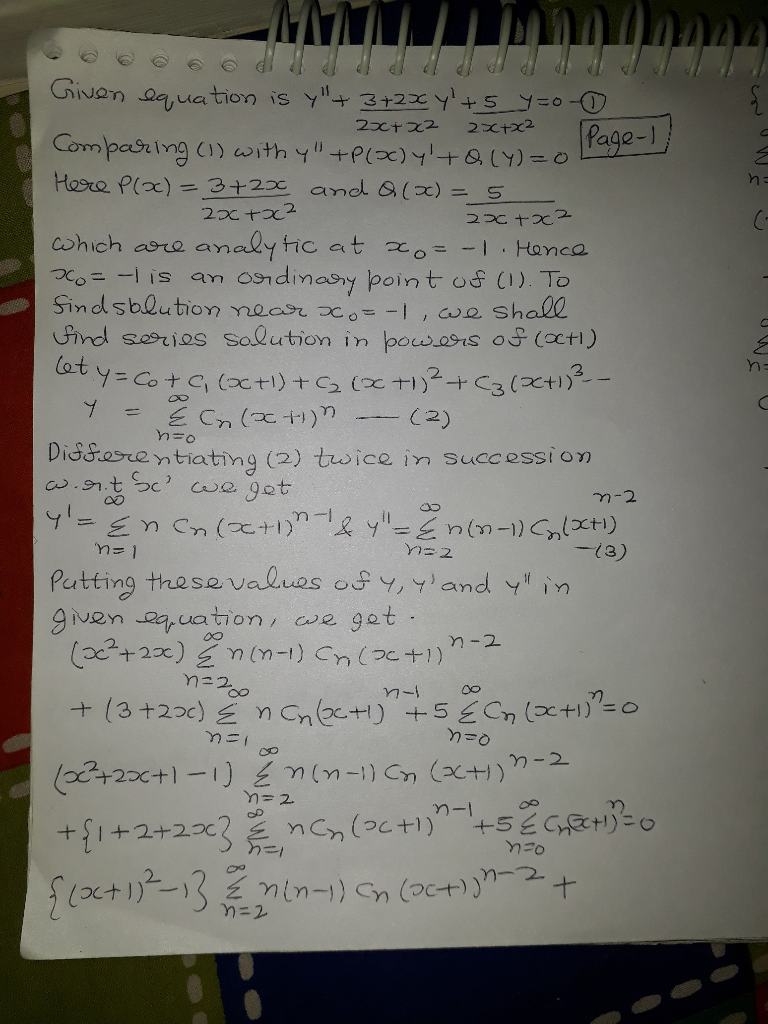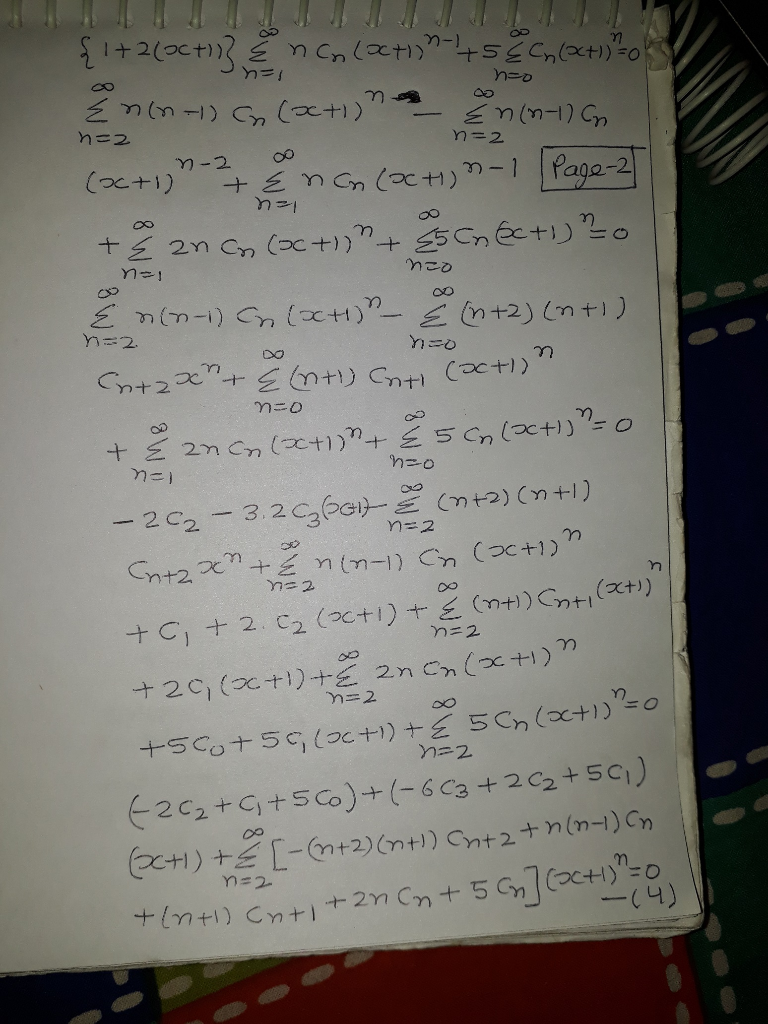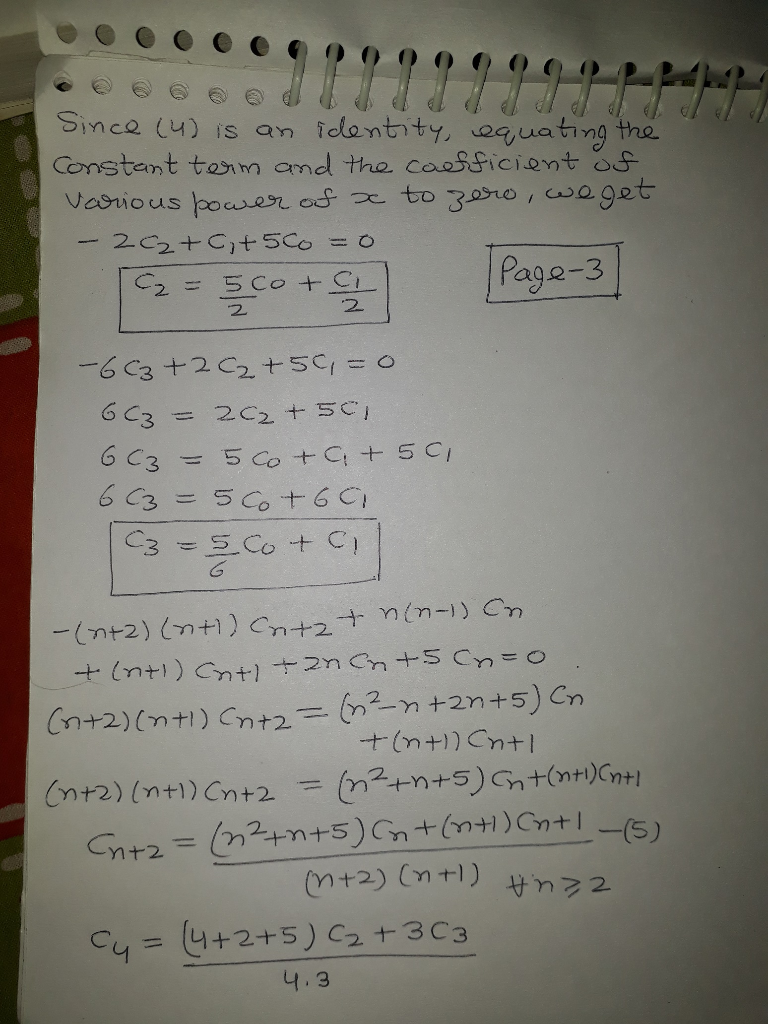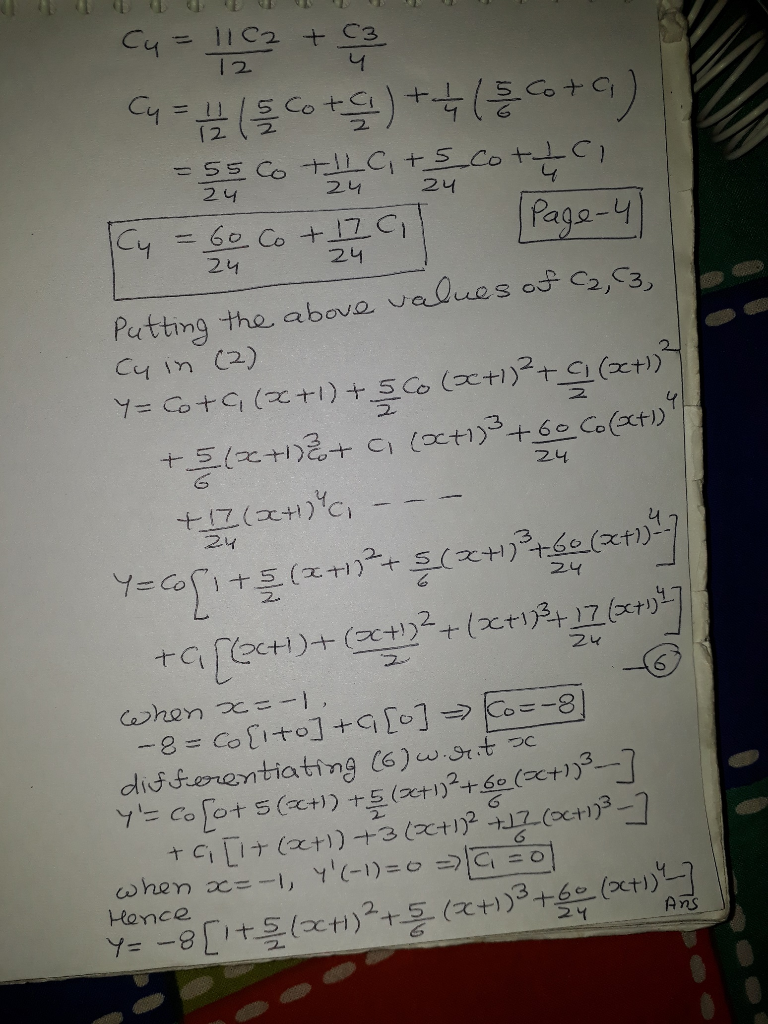##### Add Answer of: It’s review question, I need this as soon as possible. Thank you 3) For thè diferential equation: (a) The point zo =-1 is an ordinary point. Compute the recursion formula for the coefficients of...
Similar Homework Help Questions
• ### B only It’s review question, I need this as soon as possible. Thank you 3) Pol the diferential equation: (a) The point o -1 is an ordinary point. Compute the recursion formula for the coeffici...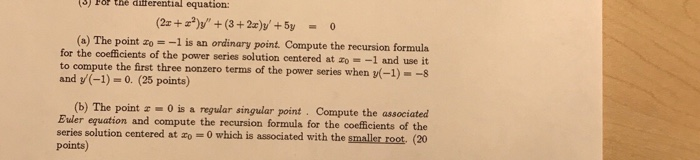B only It’s review question, I need this as soon as possible. Thank you 3) Pol the diferential equation: (a) The point o -1 is an ordinary point. Compute the recursion formula for the coefficients of the power series solution centered at zo- -1 and use it to compute the first three nonzero terms of the power series when (-1)--s and y(-1)-0. (25 points) (b) The point 0 is a regular singular point Compute the associated Buler equation and compute...

• ### 3) Pol the diferential equation: (a) The point o -1 is an ordinary point. Compute the recursion formula for the coefficients of the power series solution centered at zo- -1 and use it to compute...3) Pol the diferential equation: (a) The point o -1 is an ordinary point. Compute the recursion formula for the coefficients of the power series solution centered at zo- -1 and use it to compute the first three nonzero terms of the power series when (-1)--s and y(-1)-0. (25 points) (b) The point 0 is a regular singular point Compute the associated Buler equation and compute the recursion formula for the coefficients of the series solution centered at o 0...

• ### (6) (18 points) For the differential equation: Compute the recursion formula for the coefficients...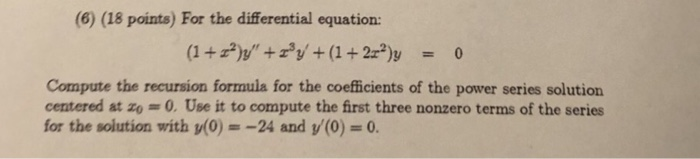(6) (18 points) For the differential equation: Compute the recursion formula for the coefficients of the power series solution centered at zo 0.Use it to compute the first three nonzero terms of the series for the solution with y(0) =-24 and y(0) = 0. (6) (18 points) For the differential equation: Compute the recursion formula for the coefficients of the power series solution centered at zo 0.Use it to compute the first three nonzero terms of the series for the...

• ### (4) (12 points)For the differential equation: Compute the recursion formula for the coefficients of the power series solution centered at o 0 and use it to compute the first three nonzero terms of...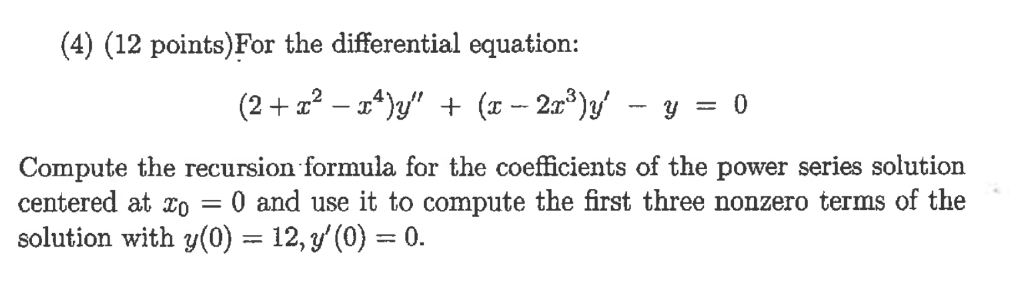(4) (12 points)For the differential equation: Compute the recursion formula for the coefficients of the power series solution centered at o 0 and use it to compute the first three nonzero terms of the solution with y(0) 12, y'(0)0. (5) (12 points)For the equation y" - 5ty -7y 0 (t>0), (t)t is a solution (a) Use the method of Reduction of Order to obtain a second, independent solution. (b) Solve the equation directly, using that it is an Euler Equation....

• ### 1) Show that zo-0 is a regular singular point for the diferenta equation Zo = 0 is a regular sing...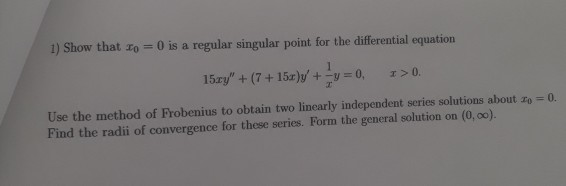please show the recurrence formula 1) Show that zo-0 is a regular singular point for the diferenta equation Zo = 0 is a regular singular point for the differential equation 15ェy" + (7 + 15r)y, +-y = 0, x>0. Use the method of Frobenius to obtain two linearly independent series solutions about zo Find the radii of convergence for these series. Form the general solution on (0, 0o). 0. 1) Show that zo-0 is a regular singular point for the...

• ### (1 point) Find a lower bound for the radius of convergence of any series solution centered at Zo = 0 for (z2-13z + 30)y" + y' + y = 0 8 Repeat the previous question for any series solution ce...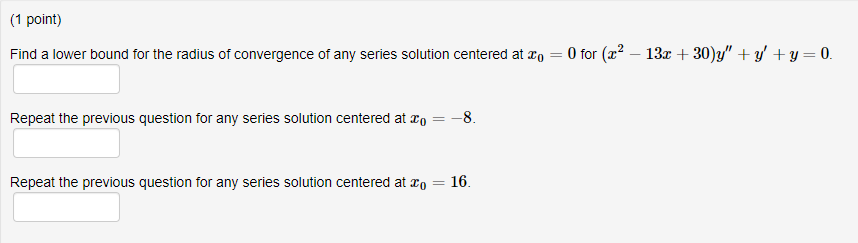(1 point) Find a lower bound for the radius of convergence of any series solution centered at Zo = 0 for (z2-13z + 30)y" + y' + y = 0 8 Repeat the previous question for any series solution centered at 20 Repeat the previous question for any series solution centered at o 16 (1 point) Find a lower bound for the radius of convergence of any series solution centered at Zo = 0 for (z2-13z + 30)y" + y'...

• ### The Legendre equation of order p is, a) Find the associated Euler equation and the characteristic equation for x = 1. b...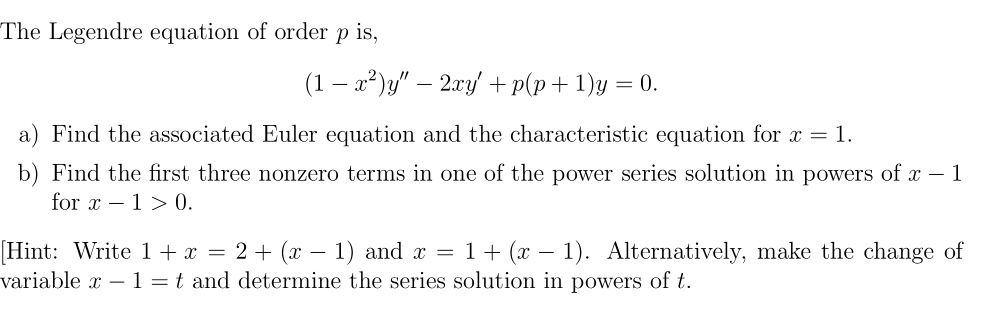The Legendre equation of order p is, a) Find the associated Euler equation and the characteristic equation for x = 1. b) Find the first three nonzero terms in one of the power series solution in powers of r -1 for x-10 Hint: Write 1 + x 2 + (2-1) and x = 1 + (x-1). Alternatively, make the change of variable x- 1-t and determine the series solution in powers of t. The Legendre equation of order p is,...

• ### Differential Equation: Series Solutions Near an Ordinary point, find a0 to a7 in the power series for the solution of the IVP.   In Exercises 19-28 find the coefficients ao. an for N at least 7 in th...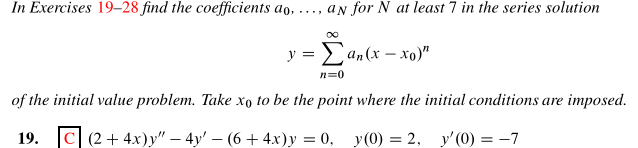Differential Equation: Series Solutions Near an Ordinary point, find a0 to a7 in the power series for the solution of the IVP.   In Exercises 19-28 find the coefficients ao. an for N at least 7 in the series solution n=0 of the initial value problem. Take xo to be the point where the initial conditions are imposed In Exercises 19-28 find the coefficients ao. an for N at least 7 in the series solution n=0 of the initial value problem....

• ### 0 of the differential equation (1 point) Find the indicated coefficients of the power series solution...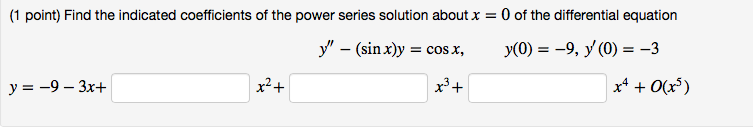0 of the differential equation (1 point) Find the indicated coefficients of the power series solution about x = У' — (sin x)y У(0) %3D —9, У (0) 3 —3 =COS X x2+ у%3 —9 — 3х+ x4O(x5) 0 of the differential equation (1 point) Find the indicated coefficients of the power series solution about x = У' — (sin x)y У(0) %3D —9, У (0) 3 —3 =COS X x2+ у%3 —9 — 3х+ x4O(x5)

• ### (1 point) Find the indicated coefficients of the power series solution about x = 0 of the differential equation -(sinx)...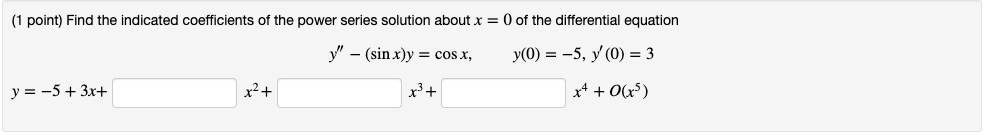(1 point) Find the indicated coefficients of the power series solution about x = 0 of the differential equation -(sinx)y y(0) = -5, y'(0) = 3 = cos x, x2 y 53x (1 point) Find the indicated coefficients of the power series solution about x = 0 of the differential equation -(sinx)y y(0) = -5, y'(0) = 3 = cos x, x2 y 53x

Need Online Homework Help?